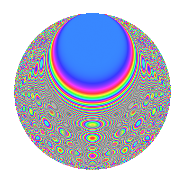# Properties

 Label 2304.2.vLevel $2304$ Weight $2$ Character orbit 2304.v Rep. character $\chi_{2304}(289,\cdot)$ Character field $\Q(\zeta_{8})$ Dimension $152$ Sturm bound $768$

# Related objects

## Defining parameters

 Level: $$N$$ $$=$$ $$2304 = 2^{8} \cdot 3^{2}$$ Weight: $$k$$ $$=$$ $$2$$ Character orbit: $$[\chi]$$ $$=$$ 2304.v (of order $$8$$ and degree $$4$$) Character conductor: $$\operatorname{cond}(\chi)$$ $$=$$ $$32$$ Character field: $$\Q(\zeta_{8})$$ Sturm bound: $$768$$

## Dimensions

The following table gives the dimensions of various subspaces of $$M_{2}(2304, [\chi])$$.

Total New Old
Modular forms 1664 168 1496
Cusp forms 1408 152 1256
Eisenstein series 256 16 240

## Trace form

 $$152q - 8q^{5} + O(q^{10})$$ $$152q - 8q^{5} + 8q^{13} - 8q^{25} - 8q^{29} + 8q^{37} + 8q^{41} - 40q^{53} + 72q^{61} + 16q^{65} - 8q^{73} - 40q^{77} - 32q^{85} + 8q^{89} - 16q^{97} + O(q^{100})$$

## Decomposition of $$S_{2}^{\mathrm{new}}(2304, [\chi])$$ into newform subspaces

The newforms in this space have not yet been added to the LMFDB.

## Decomposition of $$S_{2}^{\mathrm{old}}(2304, [\chi])$$ into lower level spaces

$$S_{2}^{\mathrm{old}}(2304, [\chi]) \cong$$ $$S_{2}^{\mathrm{new}}(32, [\chi])$$$$^{\oplus 12}$$$$\oplus$$$$S_{2}^{\mathrm{new}}(96, [\chi])$$$$^{\oplus 8}$$$$\oplus$$$$S_{2}^{\mathrm{new}}(128, [\chi])$$$$^{\oplus 6}$$$$\oplus$$$$S_{2}^{\mathrm{new}}(256, [\chi])$$$$^{\oplus 3}$$$$\oplus$$$$S_{2}^{\mathrm{new}}(288, [\chi])$$$$^{\oplus 4}$$$$\oplus$$$$S_{2}^{\mathrm{new}}(384, [\chi])$$$$^{\oplus 4}$$$$\oplus$$$$S_{2}^{\mathrm{new}}(768, [\chi])$$$$^{\oplus 2}$$$$\oplus$$$$S_{2}^{\mathrm{new}}(1152, [\chi])$$$$^{\oplus 2}$$

## Hecke characteristic polynomials

There are no characteristic polynomials of Hecke operators in the database